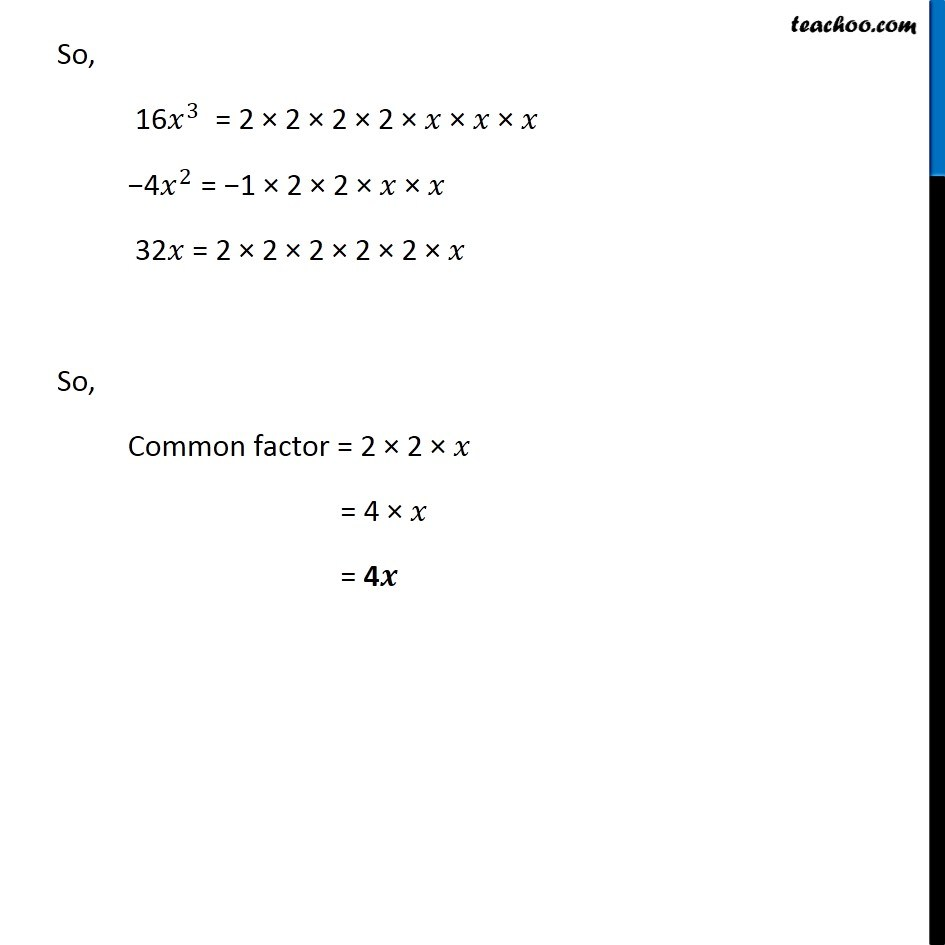Factorisation using common factors

Chapter 12 Class 8 Factorisation
Concept wiseLearn in your speed, with individual attention - Teachoo Maths 1-on-1 Class

### Transcript

Ex 12.1, 1 Find the common factors of the given terms. (vi) 〖16𝑥〗^3,−〖4𝑥〗^2, 32𝑥 16𝑥^3 = 16 × 𝑥^3 = 2 × 2 × 2 × 2 × 𝑥^3 = 2 × 2 × 2 × 2 × 𝑥 × 𝑥 × 𝑥 −4𝑥^2 = −4 × 𝑥^2 = −1 × 4 × 𝑥^2 = −1 × 2 × 2 × 𝑥^2 = −1 × 2 × 2 × 𝑥 × 𝑥 32𝑥 = 32 × 𝑥 = 2 × 2 × 2 × 2 × 2 × 𝑥 So, 16𝑥^3 = 2 × 2 × 2 × 2 × 𝑥 × 𝑥 × 𝑥 −4𝑥^2 = −1 × 2 × 2 × 𝑥 × 𝑥 32𝑥 = 2 × 2 × 2 × 2 × 2 × 𝑥 So, Common factor = 2 × 2 × 𝑥 = 4 × 𝑥 = 4𝒙